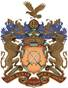••••MathsOur Vision Statement

"To provide an outstanding learning environment which challenges us to achieve our full potential and to continually share our knowledge and skills with the local and global community"

Philosophy

At Guru Nanak Sikh Acdemy we aim to provide a learning atmosphere which gives pupils the opportunity and confidence to discuss, investigate and enjoy mathematics and so develop into successful mathematicians in their adult life.

Aims

Using the New National Curriculum for our planning the children will be given the opportunity to:

• think for themselves, take responsibility for their own learning and achieve a good level of numeracy
• develop an understanding of mathematical vocabulary and notation
• develop mathematical concepts in real situations through handling materials, discussion and practical situations
• have the confidence to apply the knowledge and experience they have gained to other mathematical tasks
• have an appreciation of the logical aesthetic aspects of mathematics, through problem solving and investigation, linking this with other areas of the curriculum.

Objectives

By the end of Year 6 all pupils should:

• have a sense of the size of a number and where it fits into the number system
• know by heart number facts such as number bonds, multiplication tables, doubles and halves
• calculate accurately and efficiently, both mentally and with pencil and paper, and draw on a range of calculation strategies
• recognise when it is appropriate to use a calculator or other mathematical apparatus, and be able to do so effectively
• make sense of number problems, including non-routine problems, and recognise the operations needed to solve them
• explain their methods and reasoning using correct mathematical terms
• judge whether their answers are reasonable and have strategies for checking them where necessary
• suggest suitable units for measuring and make sensible estimates of measurements
• compare and classify shapes; identify, compare and order angles; identify lines of symmetry in 2-D shapes
• use coordinates to describe positions on a 2-D grid; describe movements between positions; plot specified points and draw sides to complete a polygon
• explain and make predictions from the numbers in graphs, diagrams, charts and tables
• draw on a range of problem solving skills to tackle problems
• recognise the links between tasks completed in class and real-life problems